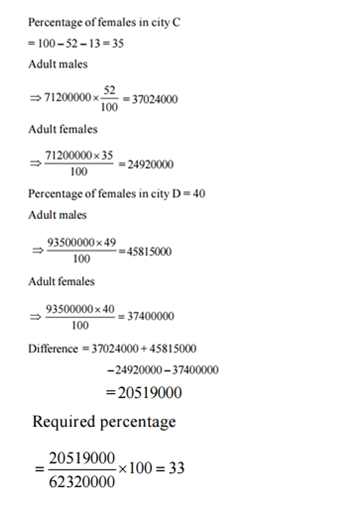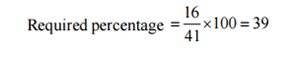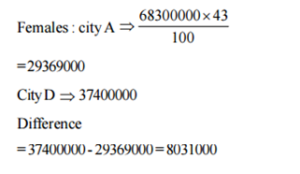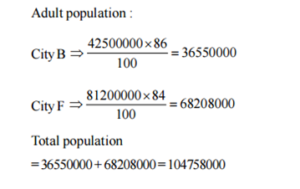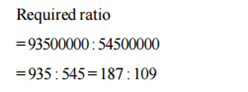# “20-20” Quantitative Aptitude | Crack SBI Clerk 2018 Day-36

Dear Friends, SBI Clerk 2018 Notification has been released we hope you all have started your preparation. Here we have started New Series of Practice Materials specially for SBI Clerk 2018. Aspirants those who are preparing for the exams can use this “20-20” Quantitative Aptitude Questions.

## Daily Practice Test Schedule | Good Luck

 Topic Daily Publishing Time Daily News Papers & Editorials 8.00 AM Current Affairs Quiz 9.00 AM Logical Reasoning 10.00 AM Quantitative Aptitude “20-20” 11.00 AM Vocabulary (Based on The Hindu) 12.00 PM Static GK Quiz 1.00 PM English Language “20-20” 2.00 PM Banking Awareness Quiz 3.00 PM Reasoning Puzzles & Seating 4.00 PM Daily Current Affairs Updates 5.00 PM Data Interpretation / Application Sums (Topic Wise) 6.00 PM Reasoning Ability “20-20” 7.00 PM English Language (New Pattern Questions) 8.00 PM General / Financial Awareness Quiz 9.00 PM

[WpProQuiz 1413]

Click “Start Quiz” to attend these Questions and view Explanation

### Click Here for SBI Clerk Prelims and Mains 2018 – Full Length Mock Test

Directions (Q. 1-5): What will come in place of question mark (?) in the given number series?

1. 65, 62.5, 67.5,60, ?
1. 70
2. 75
3. 72.5
4. 69.5
5. None of these
1. 2520, 420, 84, 21, ?
1. 15
2. 12
3. 7
4. 9
5. None of these
1. 88, 115, 145, 180, 222, ?
1. 261
2. 343
3. 216
4. 512
5. None of these
1. 17, 22, 47, 172, ? , 3922
1. 356
2. 782
3. 1187
4. 797
5. None of these
1. 56, 28, 28, ? , 224, 1792
1. 62
2. 56
3. 78
4. 42
5. None of these
1. In how many different ways can the letters of the word “INTEGRATION” be arranged so that the vowels always come together?
1. 75600
2. 66700
3. 72800
4. 56800
5. None of these
1. A, B and C started a business with their investments in the ratio of 1:2:3. After 6 months, A invested the same amount as before and B and C withdraw half of their investments. The ratio of their profits at the end of the year is?
1. 3:2:5
2. 1:5:7
3. 2:2:3
4. 4:5:5
5. None of these
1. 15 men can complete the work in 8 days. After 3 days of the work, 3 men left the job. In how many days will all of them together complete the remaining work?
1. 5 days
2. 7 ½ days
3. 8 days
4. 6 ¼ days
5. 10 days
1. If the simple interest on a certain sum of money for 4 years at 6% per annum is Rs. 240. Find the corresponding C.I?
1. 335.56784
2. 262.47696
3. 145.77834
4. 257.27896
5. None of these
1. A student gets 20% marks and fails by 60 marks. Another student gets 32% marks so he gets 84 marks more than the passing marks. Find the passing Percentage?
1. 35%
2. 20 %
3. 40 %
4. 50%
5. None of these

Directions (Q. 11-15):What should come in place of question mark (?) in the following questions?

1. 162 % of 238 + x% of 500 =2615 + √7921
1. 463.688
2. 512.788
3. 344.588
4. 656.488
5. None of these
1. 5/7 of 3/8 of (2+ (15/7) ÷ (45/49)) +50% of 450 = x- 222
1. 615.46
2. 830.26
3. 710.16
4. 520.36
5. None of these
1. (160 × 0.40)3 ÷ (928 ÷ 58)7 × (32 × 8)9 = 4? + 3
1. 22
2. 15
3. 18
4. 28
5. None of these
1. (3/8) of (16/9) of (6/15) of 45% of 4850 = ?
1. 448
2. 582
3. 678
4. 346
5. None of these
1. 242 × 6 ÷ 12 + 83 + 91 = (?)3 – 837
1. 12
2. 14
3. 13
4. 17
5. 15

Directions (Q. 16-20): In the following table, populations of 6 cities have been given. Study the following table carefully and answer the questions.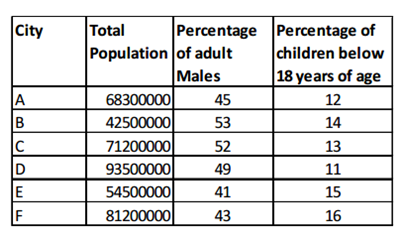1. By what percent is the number of adult males in cities C and D more than that of adult females in the same cities(Approx)?
1. 33%
2. 37%
3. 45%
4. 56%
5. 40%
1. The number of children in city F is what per cent of the number of adult females in the same city (approx)?
1. 35
2. 39
3. 42
4. 43
5. 44
1. The difference between the number of adult females in the cities A and D is?
1. 8031000
2. 8301000
3. 8130000
4. 8231000
5. None of these
1. What is the adult population in cities B and F?
1. 107458000
2. 104758000
3. 104458000
4. 114458000
5. None of these
1. What is ratio of total population of city D and city E?
1. 187 : 109
2. 187 : 119
3. 119 : 187
4. 129 : 187
5. None of these

Directions (Q. 1-5):

The pattern is, – (2.5*1), + (2.5*2), – (2.5*3), + (2.5*4)

The pattern is, ÷ 6, ÷ 5, ÷ 4, ÷ 3,..

The difference of difference is, 3, 5, 7, 9,..

The pattern is, 5, 52, 53, 54,..

The pattern is, *0.5,*1, *2, *4, *8,..

5 vowels and 6 consonants are there in the above word, Vowels always come together, So we take that as, 7!

Total ways is,

= > (7!/2!*2!) * (5!/2!)

= > 75600

Explanation:

The ratio of Profit= 1*(6) + 2*(6) : 2*(6) + 1*(6) : 3* (6) + (3/2)*(6)

= > 18 : 18 : 27

= > 2 : 2 : 3

Explanation:

Total work= 15*8 = 120 work

3 days work= 15*3 =45 work

Remaining work= 120 -45 = 75 work

Required days = 75/12 = 6 ¼ days

Explanation:

(P*4*6)/100 =240

P = 1000

Corresponding C.I,

C.I = P(1+(r/100)4) – P

= > 1000(1+(6/100)4) -1000

= > 1262.47696 -1000

= >262.47696

Let total marks be x,

(20/100)*x + 60 = (32/100)x -84

(12/100)x = 144

X= 1200

Passing mark= (20/100)*1200 +60 =300

Passing % = (300/1200)*100 = 25%

Directions (Q. 11-15):

(162*238)/100 + 5x = 2615 +89

5x= 2615+89 -385.56

5x= 2318.44 /5

X= 463.688

(5/7)*(3/8)*(13/3) +225 = x-484

65/56 +709 = x

X= 1.16 +709 =710.16

643 ÷ 167 × 2569 = 4x + 3

49 ÷ 414 × 436 = 4x + 3

49 -14 +36 = 4x + 3

31 = x+3

X=28

= > (3/8) * (16/9) * (6/15) * (45/100) * 4850

= > (2 * 45 * 97) / 15

= > 582

(24 × 24 × 6)/12 +512 +91 +837 = x3

288 +512 +91 +837 = x3

x3 = 1728

x = 12

Directions (Q. 16-20):